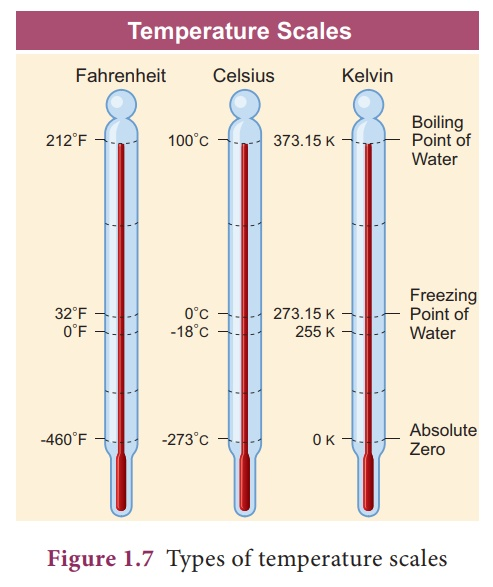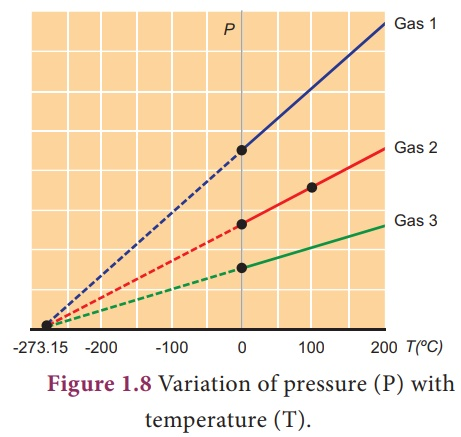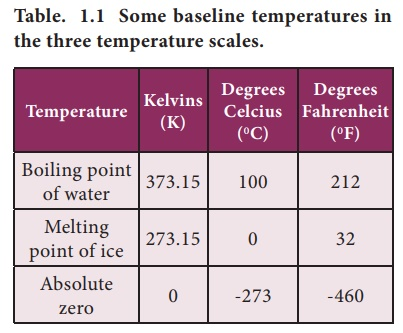Home | | Science 9th std | Concept of temperature

# Concept of temperature

Temperature is the degree of hotness or coolness of a body. The hotter the body is higher is its temperature.

Concept of temperature

Temperature is the degree of hotness or coolness of a body. The hotter the body is higher is its temperature.

## 1. Unit of Temperature

The SI unit of temperature is kelvin (K). For day to day applications, Celsius (˚C) is used. Temperature is measured with a thermometer.

## 2. Temperature scales

There are three scales of temperature.

i.               Fahrenheit scale

iii.               Kelvin or Absolute scale### i. Fahrenheit scale

In Fahrenheit scale, 32 ˚F and 212 ˚F are the freezing point and boiling points respectively. Interval has been divided into 180 parts.

### ii. Celsius temperature scale

In Celsius scale, also called centigrade scale, 0˚C and 100 ˚C are the freezing point and boiling respectively. Interval has been divided into 100 parts. The formula for converting a Celsius scale to Fahrenheit scale is:The formula for converting a Fahrenheit scale to Celsius scale is:### iii. Kelvin scale (Absolute scale)

Kelvin scale is known as the absolute scale. On the Kelvin scale 0 K represents absolute zero, the temperature at which the molecules of a substance have their lowest possible energy. The solid, liquid, gaseous phases of water can coexist in equilibrium at 273.16 K.

Kelvin is defined as 1/273.16 of the triple point temperature.

The formula for converting a Celsius scale to Kelvin scale is:

K = C + 273.15

The formula for converting a Kelvin scale to Celsius scale is:

C = K - 273.15

Absolute zero

The temperature at which the pressure and volume of a gas theoretically reaches zero is called absolute zero. This is shown in Figure 1.8.For all gases, the pressure extrapolates to zero at the temperature -273.15 ˚C. It is known as absolute zero or 0 K. Some base line temperatures in the three temperature scales are shown in Table 1.1.### Convert the following

i. 25 ºC to Kelvin

ii. 200 K to ºC

### Solution:

i. (TK) = (T°C) + 273.15

(TK) = 25 + 273.15 = 298.15 K

ii. (T°C) = (TK) - 273.15

(T°C) = 200 - 273.15 = -73.15 ºC

### Exercise 1.2

Convert the following

i. 35°C to Fahrenheit (°F)

ii. 14° F to °C

### Solution:

i. T(°F) = T(°C) × 1.8 + 32

T(°F) = 25° C × 1.8 + 32 = 77° F

ii. T(°C) = (T(°F) – 32)/ 1.8

T(°C) = (14°F – 32)/ 1.8 = -10° C

Study Material, Lecturing Notes, Assignment, Reference, Wiki description explanation, brief detail
9th Science : Heat : Concept of temperature |